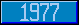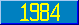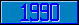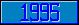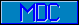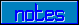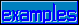## Scanning Algortitm (Pascal-like)

### Transition Diagram Scanning Algorithm (Pascal-like)

```
TYPE alpha = ARRAY[1..16] OF char;

VAR linact, comact : Boolean; case : char;

FUNCTION traversediagram(diagramname : alpha) : integer;

TYPE exitnode = (X,Y,Z,W);

VAR oneprimitivetraversed, continuescanning, pathtaken, pathexists : Boolean;

exittype : integer;

BEGIN

|   oneprimitivetraversed := FALSE;

|   continuescanning := TRUE;

|   WHILE continuescanning DO

|       BEGIN

|       |   pathtaken := FALSE;

|       |   pathexists := TRUE;

|       |   WHILE pathexists DO

|       |   |   BEGIN

|       |   |   |   find next path from this syntax node with a primitive symbol on it;

|       |   |   |   IF such a path found THEN

|       |   |   |   |   BEGIN

|       |   |   |   |   |   IF character on the path = character in the window THEN

|       |   |   |   |   |       BEGIN

|       |   |   |   |   |       |   exittype := 1;

|       |   |   |   |   |       |   oneprimitivetraversed TRUE;

|       |   |   |   |   |       |   mark this path as the chosen path to be taken;

|       |   |   |   |   |       |   move window one position to the right;

|       |   |   |   |   |       |   IF input string is exhausted THEN syntax_ error

|       |   |   |   |   |       |   ELSE BEGIN pathtaken := TRUE; pathexists FALSE; END

|       |   |   |   |   |       END

|       |   |   |   |   END

|       |   |   |   ELSE pathexists := FALSE;

|       |   |   END;

|       |   IF ¬ pathtaken THEN

|       |   |   BEGIN

|       |   |   |   pathexists := TRUE;

|       |   |   |   WHILE pathexists DO

|       |   |   |       BEGIN

|       |   |   |       |   find next path from this syntax node with a call to "diagramname";

|       |   |   |       |   IF such a path found THEN

|       |   |   |       |   |   BEGIN

|       |   |   |       |   |   |   exittype := traversediagram("diagramname");

|       |   |   |       |   |   |   IF exittype ≠ 0 THEN

|       |   |   |       |   |   |       BEGIN

|       |   |   |       |   |   |       |   pathtaken := TRUE;

|       |   |   |       |   |   |       |   pathexists := FALSE;

|       |   |   |       |   |   |       |   mark this path as the chosen path to be taken;

|       |   |   |       |   |   |       END

|       |   |   |       |   |   END

|       |   |   |       |   ELSE pathexists := FALSE;

|       |   |   |       END

|       |   |   END;

|       |   IF ¬ (pathtaken) ∧ there is a blank path from this syntax node THEN

|       |   |   BEGIN

|       |   |   |   exittype := 1;

|       |   |   |   pathtaken := TRUE;

|       |   |   |   mark this path as the chosen path to be taken;

|       |   |   END;

|       |   IF pathtaken THEN

|       |   |   BEGIN

|       |   |   |   CASE exittype OF

|       |   |   |   |   1: case := 'X';

|       |   |   |   |   2: case := 'Y';

|       |   |   |   |   3: case := 'Z';

|       |   |   |   |   4: case := 'W';

|       |   |   |   END;

|       |   |   |   find marked path from this syntax node and move to next syntax node along it;

|       |   |   |   IF path traversed specified an action THEN

|       |   |   |   |   BEGIN

|       |   |   |   |   |   IF (linact ∧ comact) ∨ action is a privileged action THEN DO action;

|       |   |   |   |   END;

|       |   |   |   IF next syntax node is an exitnode THEN

|       |   |   |       BEGIN

|       |   |   |       |   continuescanning := FALSE;

|       |   |   |       |   CASE exitnode OF

|       |   |   |       |   |   Z: traversediagram := 1;

|       |   |   |       |   |   Y: traversediagram := 2;

|       |   |   |       |   |   Z: traversediagram := 3;

|       |   |   |       |   |   W: traversediagram := 4;

|       |   |   |       |   END

|       |   |   |       END

|       |   |   END

|       |   ELSE

|       |       BEGIN

|       |       |   IF oneprimitivetraversed THEN syntax error

|       |       |   ELSE

|       |       |       BEGIN

|       |       |       |   traversediagram := 0;

|       |       |       |   continuescanning := FALSE;

|       |       |       END

|       |       END

|       END

END

```